# Test: Theory of Random Variable & Noise

## 10 Questions MCQ Test GATE ECE (Electronics) 2023 Mock Test Series | Test: Theory of Random Variable & Noise

Description
Attempt Test: Theory of Random Variable & Noise | 10 questions in 30 minutes | Mock test for GATE preparation | Free important questions MCQ to study GATE ECE (Electronics) 2023 Mock Test Series for GATE Exam | Download free PDF with solutions
QUESTION: 1

### A certain random variable has the CDF (cumulative distribution function) given by: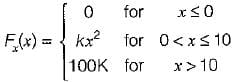Which of the following options is/are not correct?

Solution: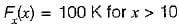Since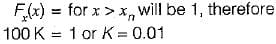Now,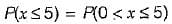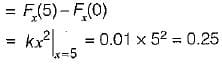Also,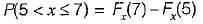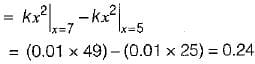Hence, option (c) is not correct.

QUESTION: 2

### A radio receiver with 10 kHz bandwidth has a noise figure of 30 dB. The noise power at input is (take K = 1.38 x 10-23 J/Kelvin and assume room temperature of 300 K)

Solution:

Noise figure,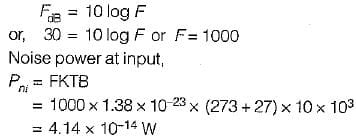QUESTION: 3

### The probability density function of a random variable is given by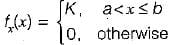The value of constant K is

Solution:

Since the area under the probability density function is equal to 1, therefore.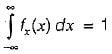or,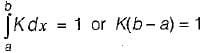or,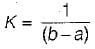QUESTION: 4

Consider the following statements associated with different types of noise occurring in nature:
1. The flicker noise occurs at high operating frequencies (few MHz).
2. Shot noise is produced due to random variations in the arrival of electrons at the output electrode of an amplifying device.
3. The thermal noise is proportional to temperature and the value of the resistance and is independent of bandwidth.
4. Noise can also be represented by fictitious temperature called noise temperature.
Which of the statements given above is/are not correct?

Solution:
• The flicker noise occurs at low operating frequencies (few KHz). Hence, statement-1 is not correct.
• The thermal noise voltage is given by,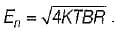Hence, it is proportional to temperature, bandwidth and the value of resistance. Thus, statement-3 is not correct.
• Statements 1 and 4 are correct.
QUESTION: 5

Over a certain binary communication channel (BCC), symbol ‘O' is transmitted with a probability of 0.4 and symbol ‘1’ is transmitted with a probability of 0.6. The probability of error when '1' is transmitted is 10-4 and that when a ‘0' is transmitted is 10-6.
What is the probability of error in the channel?

Solution:

BCC with different probabilities: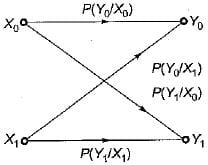According to question,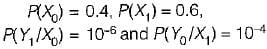Here,  P(Y0/X0) =  Probability of error when ‘1 ’ is transmitted
P(Y1/X0) = Probability of error when ‘0 ’ is transmitted
∴ Probability of error in channel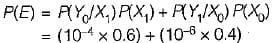or, P(E) = 0.604 x 10-4

QUESTION: 6

Thermal noise is also known as

Solution:

The electronic components generate thermal, shot noise. The thermal noise is also called Johnson noise.

QUESTION: 7

The joint PDF of the.random variable x and y is given by,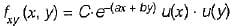where, a and b are constants. The value of C is

Solution:

The joint CDF (cumulative distribution function ) is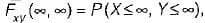which will be equal to 1 because the probability of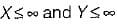covers all the possible values of random variables X and Y.
∴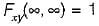According to the definition of CDF, we have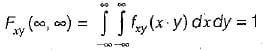Substituting the value of PDF, we have: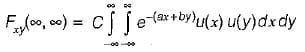or,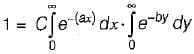or,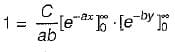or,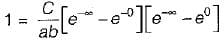or,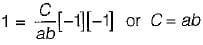QUESTION: 8

Assertion (A): if the random process x(t) changes very rapidly with time, the autocorrelation function will decrease rapidly to zero.
Reason (R): The autocorrelation function Rx(τ) is a measure of interdependence of two random variables obtained by observing the random process x(t) at time τ seconds apart.

Solution: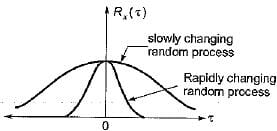From above figure we can conclude that both assertion and reason are true and reason is the correct explanation of assertion.

QUESTION: 9

Consider the following statements associated with conditional probability density function (PDF):
1. The conditional PDF is basically a probability, density function (PDF).
2. The conditional PDF is a non-negative function.
3. The area under a conditional PDF is always equal to 1,
4. The conditional density functions reduce to the marginal density functions.
Which of the statements given above are correct?

Solution:

• The conditional PDF is basically a PDF Since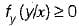i.e. the conditional PDF is a non ­negative function.
Hence, statements 1 and 2 are correct.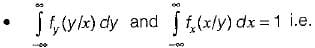th e area under a conditional PDF is always equal to 1. Hence, statement-3 is also correct.
• If the random variables x and y are statistically independent, then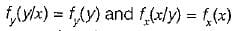This means that the conditional density functions reduce to the marginal density functions. Hence, statement-4 is also correct.

QUESTION: 10

The distribution function of random variable is

Solution:

The distribution function of a random variable is the probability that the value taken by the random variable is less than or equal to the real number x.Use Code STAYHOME200 and get INR 200 additional OFF Use Coupon Code